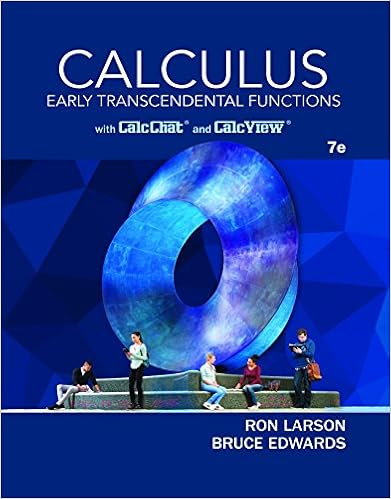# MATH1014 Tutorial Notes 03 including exercises.pdf -...

• Notes
• 10

This preview shows page 1 - 4 out of 10 pages.

##### We have textbook solutions for you!
The document you are viewing contains questions related to this textbook.The document you are viewing contains questions related to this textbook.
Chapter 7 / Exercise 17
Calculus: Early Transcendental Functions
Edwards/LarsonExpert Verified
MATH1014 Calculus II (2017 Fall) Tutorial Notes 3(Phyllis LIANG) Page 1of 5 MATH 1014 Tutorial Notes 3 Topics covered in tutorial 03: 1.Arc Length2.Area of a Surface of Revolution3.Physical Application1. Arc Length What you need to know: The Arc Length Formula The Arc Length Formula: If݂is continuous on [ܽ, ܾ], then the length of the curve ݕ= ݂ሺݔ, ܽ≤ ݔ≤ ܾ, is = ∫+[ሺ࢞ሻ]ࢊ࢞= ∫+ࢊ࢟ࢊ࢞ࢊ࢞Remark:Let ݔ= ݃ሺݕ, ܿ≤ ݕ≤ ݀have a continuous first derivative on the interval [ܿ, ݀]. The length of the curve from ܿto ݀is = ∫+[ሺ࢟ሻ]ࢊ࢟= ∫+ࢊ࢞ࢊ࢟ࢊ࢟Example 3.1Find the arc length of the function ݕ= ݂ሺݔሻ=ݔ− ʹሻover [ʹ, ͵].
##### We have textbook solutions for you!
The document you are viewing contains questions related to this textbook.The document you are viewing contains questions related to this textbook.
Chapter 7 / Exercise 17
Calculus: Early Transcendental Functions
Edwards/LarsonExpert Verified
MATH1014 Calculus II (2017 Fall) Tutorial Notes 3(Phyllis LIANG) Page 2of 5 2. Area of a Surface of RevolutionWhat you need to know: • Calculate the Area of a Surface of Revolution Area of a Surface of Revolution:
Example 3.2Find the area of the surface obtained by rotating the arc ݕ= √Ͷݔ, −ͳ≤ ݔ≤ ͳabout the x− axis.
MATH1014 Calculus II (2017 Fall) Tutorial Notes 3(Phyllis LIANG) Page 3of 5 3. Physical Application What you need to know:
The mass of an object with variable density: Suppose that a thin bar or wire is represented by a line segment on the interval [ܽ, ܾ], with density function
•••